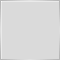# Drawing Line Start from specific point - page 2Moderator
2312

I'm not entirely sure what you're after but I'll give you a few pointers that may (or may not) help along the way.

But I think you're going to need to do quite a bit of reading...

This is just a minor point that makes your indicator more user friendly:

```//extern int   MA_Method = 0 ;          // 0=sma, 1=ema, 2=smma, 3=lwma
//extern int   MA_Price  = 0 ;          // 0=close, 1=open, 2=high, 3=low, 4=median, 5=typical, 6=weighted
extern ENUM_MA_METHOD     MA_Method = MODE_SMA;    // MA Method
extern ENUM_APPLIED_PRICE MA_Price  = PRICE_CLOSE; // Ma Applied Price
```

Let's assume the time is 14:00. Your code will retrieve the MAs as follows:

```double Current_MA_H1    = iMA(Symbol(),PERIOD_H1,MA_Period,MA_Shift,MA_Method,MA_Price,0 ), // 14:00
Previous_MA_1_H1 = iMA(Symbol(),PERIOD_H1,MA_Period,MA_Shift,MA_Method,MA_Price,1 ), // 13:00
Previous_MA_2_H1 = iMA(Symbol(),PERIOD_H1,MA_Period,MA_Shift,MA_Method,MA_Price,2 ), // 12:00
Previous_MA_3_H1 = iMA(Symbol(),PERIOD_H1,MA_Period,MA_Shift,MA_Method,MA_Price,3 ); // 11:00
```

Let's also assume this indicator is applied to the M1 chart (i.e. not the H1 chart), so the following applies.

Close = close price of 14:00 bar

Close = close price of 13:59 bar

Close = close price of 13:58 bar

Close = close price of 13:57 bar

Your triggering logic has been written

```double M1_BUY_CONDITION  = (Close < Previous_MA_3_H1 && Close > Previous_MA_2_H1 && Close > Close && Volume ==1 );
double M1_SELL_CONDITION = (Close > Previous_MA_3_H1 && Close < Previous_MA_2_H1 && Close < Close && Volume ==1 );```

So you are comparing the close at 13:57 to the H1 MA at 11:00, and the close at 13:58 to the H1 MA at 12:00 etc... is this what you intend?

Also, you are storing a bool (true or false) in a double variable. Is this what you want?

What is the purpose of Volume==1? If you are trying to detect a new bar, as a general rule use time.

You also need to be very careful with your brackets. This code works as follows:

``` if(M1_BUY_CONDITION)

ObjectSetInteger(0,M1_BUY_CONDITION,OBJPROP_RAY,false); // runs all the time
ObjectSetInteger(0,M1_BUY_CONDITION,OBJPROP_TIMEFRAMES,OBJ_PERIOD_M15|OBJ_PERIOD_H1); // runs all the time
ObjectSetInteger(0,M1_BUY_CONDITION,OBJPROP_COLOR,clrRed); // runs all the time
```

So you probably want this:

```if(M1_BUY_CONDITION)
{
}
```

You will probably also want to look up some of the functions you are using. If you press F1 from MetaEditor it will open the reference for what the cursor is currently on.

This code will only draw the line on M15 and H1 charts. Is that what you want?

`  ObjectSetInteger(0,M1_BUY_CONDITION,OBJPROP_TIMEFRAMES,OBJ_PERIOD_M15|OBJ_PERIOD_H1);`

There is also an issue with your line names. You are trying to call your lines either 0 or 1 (i.e. whatever the value of M1_BUY_CONDITION is).

Your line will also have the same start and end points. Sort of like a dot rather than a line:

`ObjectCreate(0,M1_BUY_CONDITION,OBJ_TREND,0,Time,High,Time,High);`

The whole IsDrawn approach was just to show how a line could be drawn in isolation - it wouldn't really be applicable here. I'm presuming you want the line(s) to be drawn whenever your criteria is met.251

Thank you very much and I appreciate your patience, I'm Just start to learn coding251

This is what I'm trying to do.